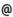## "Babes-Bolyai" University of Cluj-Napoca Faculty of Mathematics and Computer Science

 Nonsmooth methods in mathematical economics
 Code Semes-ter Hours: C+S+L Credits Type Section MO052 5 2+2+0 6 compulsory Matematici aplicate
 Teaching Staff in Charge
 Prof. MURESAN Marian, Ph.D., mmarianmath.ubbcluj.ro
 Aims Getting to know several modern results on mathematical economy with emphasis on equilibria of economical problems.
 Content I. Introduction to set-valued analysis. Multifunctions. Concepts of measurability. Selections. II. Convex analysis. Convex functions. Separation theorems. Fenchel's theorem. Conjugate functions. Support functions. III. Nonsmooth analysis. Subdiferentials. Tangent and normal cones. Fermat's theorem. IV. Two-persons games. Brouwer's fixed point theorem. Games in normal form. Pareto optima. Conservative strategies. Cournot's duopoly. V. Nonlinear equations and inclusions. The Debreu-Gale-Nikaido theorem. Tangential condition. Viability theorem. Fixed point theorems. Equilibrium of a dynamic economy. Walras's equilibrium. Stakelberg equilibrium. Variational inequalities. Welfare theorems.
 References 1. AUBIN, L.-P., Optima and Equilibria, Springer, Berlin, 1998. 2. MURESAN, M., An introduction to Set-Valued Analysis, Cluj University Press, 1999. 3. MURESAN, M., Introducere in control optimal, Risoprint, Cluj-Napoca, 1999. 4. MURESAN, M., Analiza neneteda si aplicatii, Risoprint, Cluj-Napoca, 2001. 5. PETRUSEL, A., Multi-functii si aplicatii, Presa Universitara Clujeana, Cluj-Napoca, 2002.
 Assessment Written and spoken exam. 30% from the final mark is the activity at the seminar.